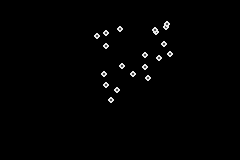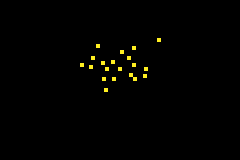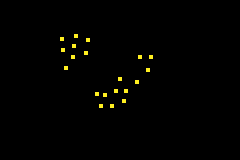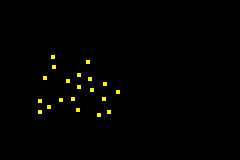[procgen]

I have had an idea for a game that needs to use a boids algorithm for a while now.

### Boids Algorithm

To put it simply Boids as a algorithm works fairly similarly to cellular automata and conways game of life. There are a few simple rules that all parties action each frame. Then positions are updated.

Have a look at the original creator of Boids explanation of it if you are curious.

But I will go through most of it as we implement it below.

To mimic flocking the rules are as follows...

#### Cohesion

The birds should stay together somewhat. To implement this, most algorithms simply move the 'boid' towards the centre of all other boids.

Having hit limits on the GBA already though, my plan here is to include the current 'boid' in this average so that we don't have to calculate a different centre for each individual 'boid'. Also the more 'boids' we have the less important this part of the calculation is.

``void CohesionRule::execute(bn::vector<Boid, 32>& boids){    //find centre of bird mass    bn::fixed_point centre = bn::fixed_point(0,0);    for(int i = 0; i < boids->size(); i++){        centre += boids->at(i).pos();    }    centre/= boids->size();    //apply to each boid    for(int i = 0; i < boids->size(); i++){        boids->at(i).add_vel((centre - boids->at(i).pos()) / _cohesion_force);    }}``

Here you can see that we have a variable `_cohesion_force`, this is used to tweak the force applied to the 'boids' to move them toward the centre of mass.

#### Separation

Boids shouldn't collide. This rule says that if you are too close to another boid, you should move away from it. Unfortunately this does mean that we have to do the calculation for each individual boid.

``void SeparationRule::execute(bn::vector<Boid, 32>& boids){    //  for each boid    for(int i = 0; i < boids->size(); i++){        //for each other boid        for(int j = 0; j < boids->size(); j++){            if( j != i){                //if near                if(bn::abs(boids->at(i).pos().x() - boids->at(j).pos().x()) < _separation_distance){                    if(bn::abs(boids->at(i).pos().y() - boids->at(j).pos().y()) < _separation_distance){                        boids->at(i).add_vel((boids->at(i).pos() - boids->at(j).pos()));                    }                }            }        }    }}``

Here `_separation_distance` is the distance at which 'boids' should avoid getting from each other, note that these forces are applied to both 'boids' in this relationship.

#### Alignment

'Boids' that are close together should fly in the same direction. Within a certain distance, 'boids' should try to align themselves with their peers.

``void AlignmentRule::execute(bn::vector<Boid, 32>& boids_ptr){    bn::vector<Boid, 32>* boids = &boids_ptr;    //  for each boid    for(int i = 0; i < boids->size(); i++){        //for each other boid        for(int j = 0; j < boids->size(); j++){            if( j != i){                if(bn::abs(boids->at(i).pos().x() - boids->at(j).pos().x()) < _alignment_distance){                    if(bn::abs(boids->at(i).pos().y() - boids->at(j).pos().y()) < _alignment_distance){                        //add the velocity of the neighbouring bird                        boids->at(i).add_vel(boids->at(j).vel());                    }                }            }        }    }}``

Here `_alignment_distance` represents how close a bird should be in order to align with it.

And what we get is something like this...Now I can only get about 10 onto the screen and still hit 60fps, the example above is at 30fps.

At this point though I think I can improve the algorithm a little and get some more on there.

### Attempt #1

There are a lot of loops over the same set of 'boids'.

Maybe we can combine all the rules into a single MEGA RULE! MUAHAHAH!

Okay. TRUTH TIME. I did this. And I tried to make it work and look good. But for some reason (I think I know the reason). It just didn't want to work for me.

Okay. The reason is that previously the alignment rule relies on all boids velocities already being updated for their cohesion and separation steps.

It may not look as pleasing, but with the new AllRule step I can get a consistent 68% CPU and 60fps for 20 boids.### Attempt #2

When I wrote the three rules originally I didn't consider performance at all. In the Alignment step I call `boids->at(i).pos()` twice for each loop of `j`. So by caching that position I can save calling this a whole heap. It now looks like this...

``void AlignmentRule::execute(bn::vector<Boid, 32>& boids){    //  for each boid    for(int i = 0; i < boids->size(); i++){        _current_pos = boids->at(i).pos();        //for each other boid        for(int j = 0; j < boids->size(); j++){            if( j != i){                _jpos = boids->at(j).pos();                if(bn::abs(_current_pos.x() - _jpos.x()) < _alignment_reach){                    if(bn::abs(_current_pos.y() - _jpos.y()) < _alignment_reach){                        boids->at(i).add_vel(boids->at(j).vel());                    }                }            }        }    }}``

With this and similar improvements I can stick to 60fps (allbeit at 90% cpu usage) for the same number of boids.### Scatter

Lastly I added a scatter rules so that I can dump a .gba here and it actually be a little interactive (press 'a'). Hint, it is actually just the opposite of the Cohesion rules.Also source code is available here.

And lastly if this has piqued your curiosity you should also look at this boids explanation, complete with psuedocode and other behaviour (like perching and avoidance).

Previous Post - Jono has a Plan
Next Post - Mapping out an MVP for Feline

Want to get notified next time I post?